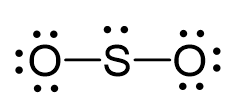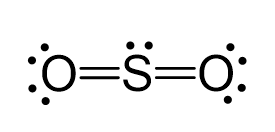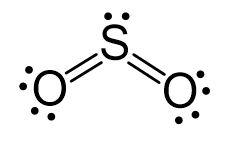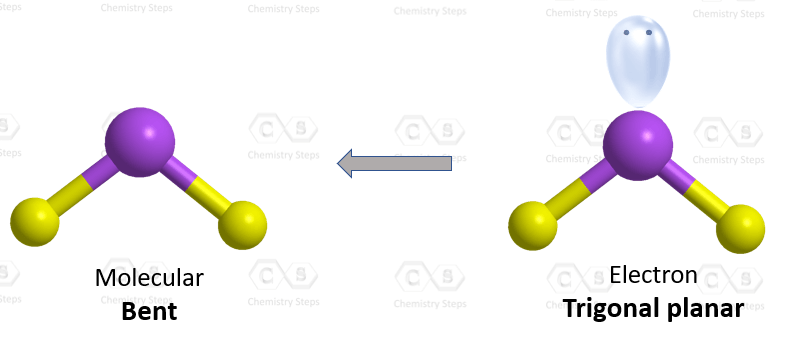## Examples

Sulfur is the central atom, so we can draw the skeletal structure:There are 3×6 = 18 electrons, and 4 of them are used to make 2 bonds. The two oxygens take 6 lone pairs, and the remaining one goes to the sulfur:As it is drawn, the problems with this structure are that the sulfur lacks an octet and the oxygens have only one bond and three lone pairs. Remember, the normal valency of oxygens is having two bonds and two lone pairs otherwise a formal charge needs to be assigned.

Therefore, one lone pair from each oxygen is used to make an additional bond with the sulfur:The central atom has a steric number of 3 – two atoms and one lone pair. The electron geometry, therefore, is trigonal planar, and the molecular geometry is bent.The steric number (the sum of the number of the atoms and lone pairs) of the sulfur is 3 which corresponds to sp2-hybridization.

More practice examples on geometry and hybridization in this multiple-choice quiz: